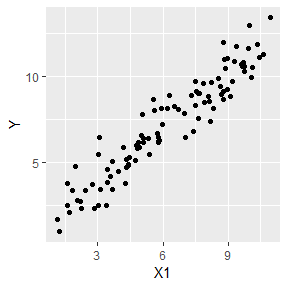# Introduction

### Package Overview

Often the first problem in understanding the generalized linear model in a practical way is finding good data. Common problems include finding data with a small number of rows, the response variable does not follow a family in the glm framework, or the data is messy and needs a lot of work before statistical analysis can begin. This package alleviates all of these by allowing you to create the data you want. With data in hand, you can empirically answer any question you have.

The goal of this package is to strike a balance between mathematical flexibility and simplicity of use. You can control the sample size, link function, number of unrelated variables, and ancillary parameter when applicable. Default values are carefully chosen so data can be generated without thinking about mathematical connections between weights, links, and distributions.

### Getting Familiar With The Package

All functions return a tibble. The only thing that changes between functions is the distribution of Y. In simulate gaussian, Y follows a gaussian distribution. In simulate_gamma, Y follows a gamma distribution. Think of these functions as helpers that create data. With this data, you can test questions and get familiar with the generalized linear model.

### Example 1: Create Textbook Data for Linear Model

library(GlmSimulatoR)
library(ggplot2)

set.seed(1)
simdata <- simulate_gaussian(N = 100, weights = 1, xrange = 10, ancillary = 1) #GlmSimulatoR function
ggplot(simdata, aes(x = X1, y = Y)) +
geom_point()rm(simdata)

### Question 1: Is A Sample Size Of 200 Enough To Get Close Estimates Of The True Weights?

set.seed(1)
simdata <- simulate_gaussian(N = 200, weights = c(1, 2, 3))

glmModel <- glm(Y ~ X1 + X2 + X3, data = simdata, family = gaussian(link = "identity"))
summary(glmModel)\$coefficients
#>              Estimate Std. Error   t value     Pr(>|t|)
#> (Intercept) 2.9138043  0.7011699  4.155633 4.843103e-05
#> X1          0.9833586  0.2868396  3.428253 7.403616e-04
#> X2          1.7882468  0.2701817  6.618683 3.386406e-10
#> X3          3.2822020  0.2640478 12.430334 1.550439e-26

rm(glmModel)

In the above, we see the estimates are close to the weights argument. The mathematics behind the generalized linear model worked well.